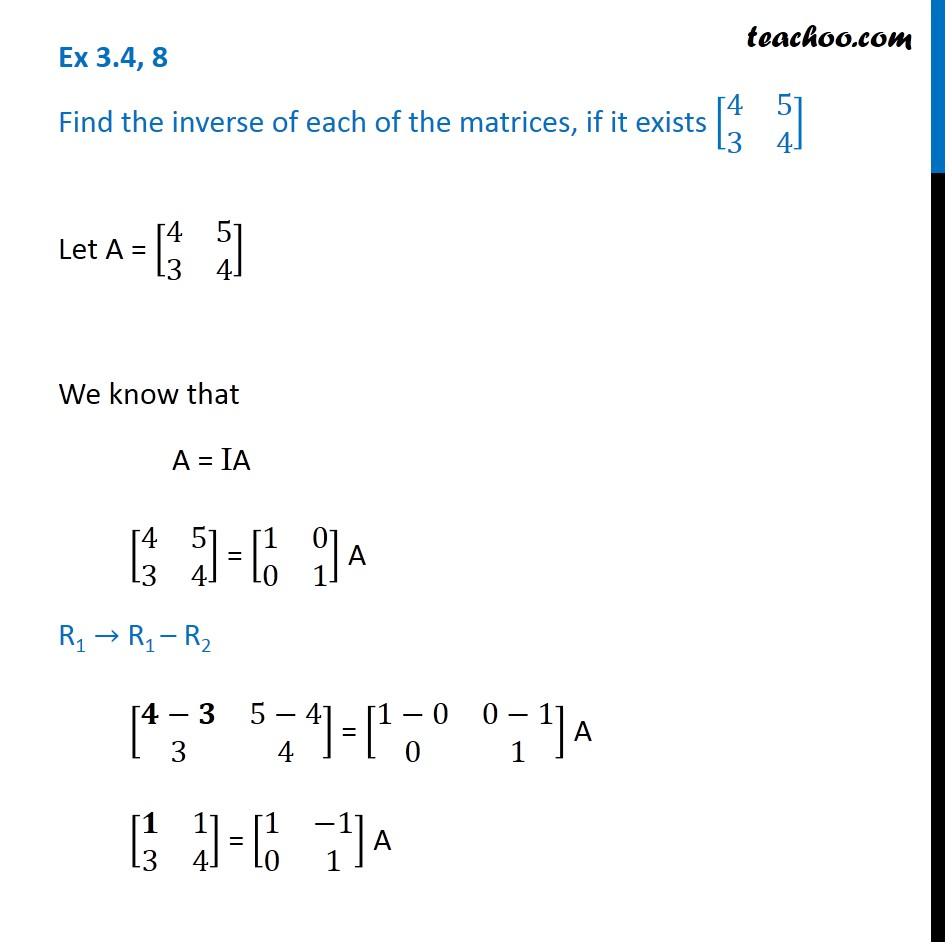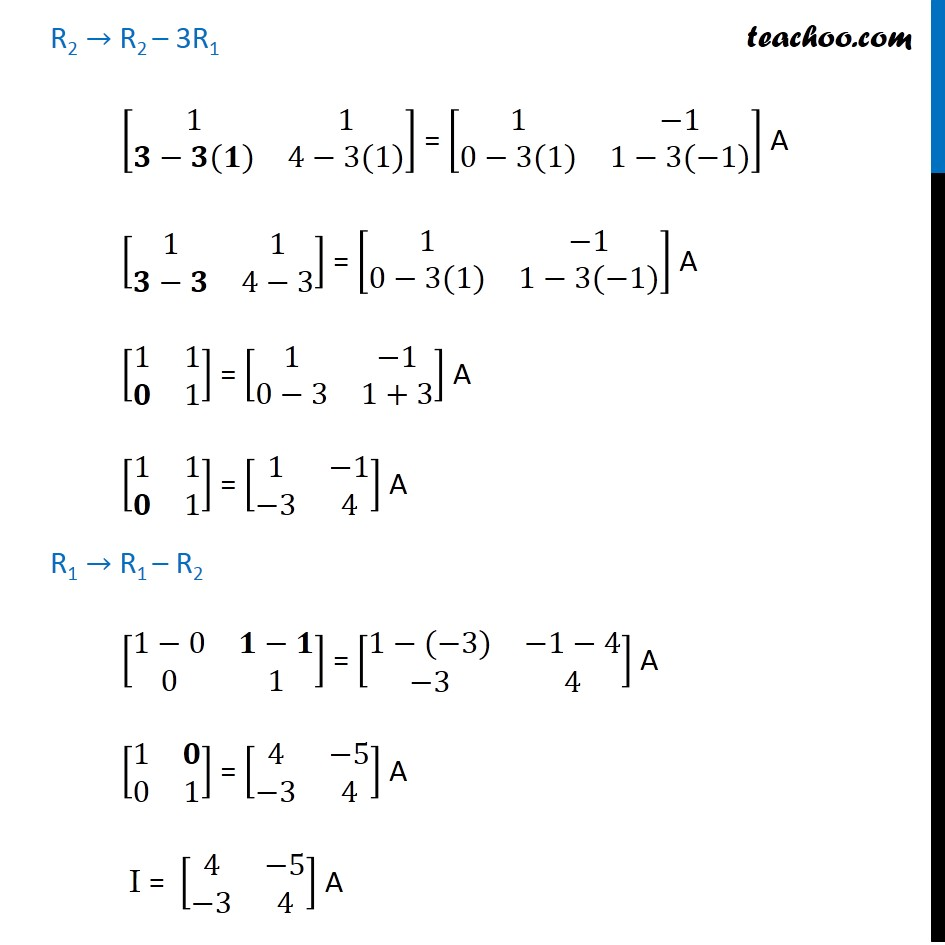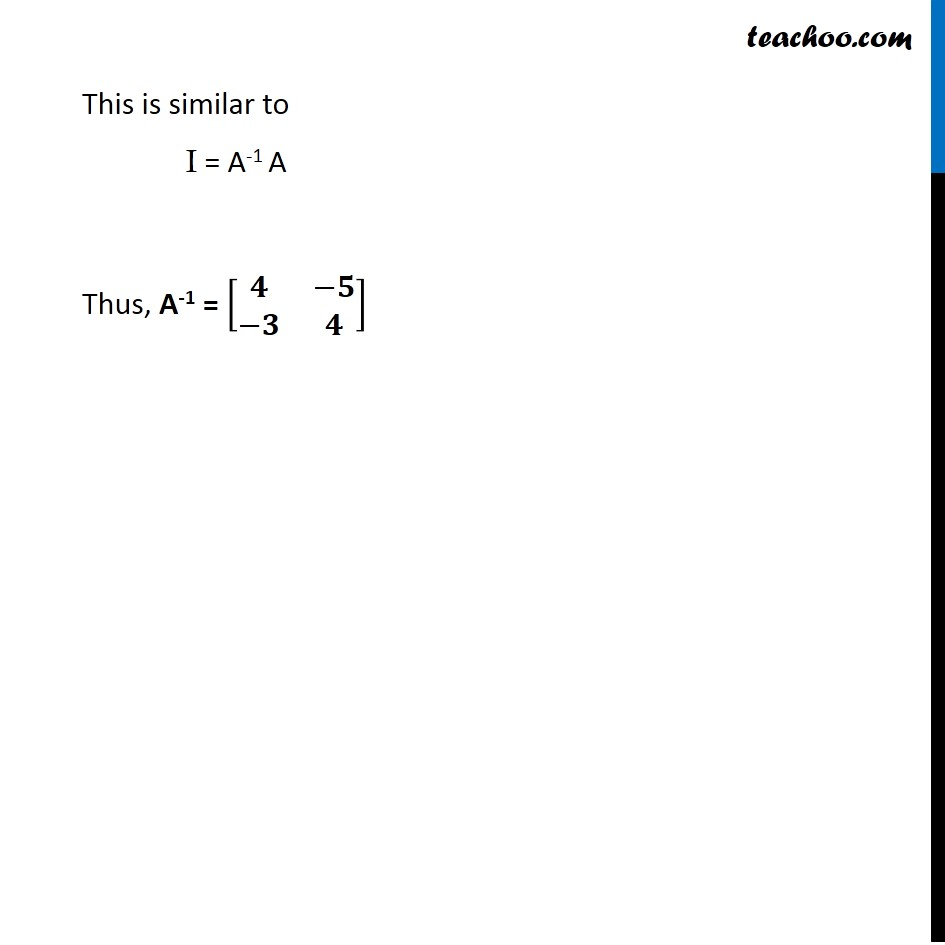Ex 3.4

Chapter 3 Class 12 Matrices
Serial order wiseLearn in your speed, with individual attention - Teachoo Maths 1-on-1 Class

### Transcript

Ex 3.4, 8 Find the inverse of each of the matrices, if it exists [■8(4&5@3&4)] Let A = [■8(4&5@3&4)] We know that A = IA [■8(4&5@3&4)] = [■8(1&0@0&1)] A R1 → R1 – R2 [■8(𝟒−𝟑&5−4@3&4)] = [■8(1−0&0−1@0&1)] A [■8(𝟏&1@3&4)] = [■8(1&−1@0&1)] A R2 → R2 – 3R1 [■8(1&1@𝟑−𝟑(𝟏)&4−3(1))] = [■8(1&−1@0−3(1)&1−3(−1))] A [■8(1&1@𝟑−𝟑&4−3)] = [■8(1&−1@0−3(1)&1−3(−1))] A [■8(1&1@𝟎&1)] = [■8(1&−1@0−3&1+3)] A [■8(1&1@𝟎&1)] = [■8(1&−1@−3&4)] A R1 → R1 – R2 [■8(1−0&𝟏−𝟏@0&1)] = [■8(1−(−3)&−1−4@−3&4)] A [■8(1&𝟎@0&1)] = [■8(4&−5@−3&4)] A I = [■8(4&−5@−3&4)] A This is similar to I = A-1 A Thus, A-1 = [■8(𝟒&−𝟓@−𝟑&𝟒)]